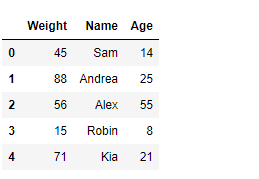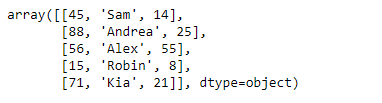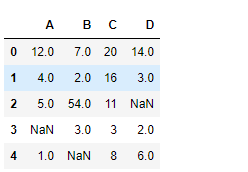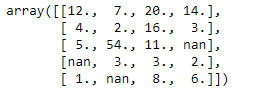Related Articles

# Python | Pandas DataFrame.values

• Last Updated : 20 Feb, 2019

Pandas DataFrame is a two-dimensional size-mutable, potentially heterogeneous tabular data structure with labeled axes (rows and columns). Arithmetic operations align on both row and column labels. It can be thought of as a dict-like container for Series objects. This is the primary data structure of the Pandas.

Pandas` DataFrame.values` attribute return a Numpy representation of the given DataFrame.

Syntax: DataFrame.values

Parameter : None

Returns : array

Example #1: Use `DataFrame.values` attribute to return the numpy representation of the given DataFrame.

 `# importing pandas as pd``import` `pandas as pd`` ` `# Creating the DataFrame``df ``=` `pd.DataFrame({``'Weight'``:[``45``, ``88``, ``56``, ``15``, ``71``],``                   ``'Name'``:[``'Sam'``, ``'Andrea'``, ``'Alex'``, ``'Robin'``, ``'Kia'``],``                   ``'Age'``:[``14``, ``25``, ``55``, ``8``, ``21``]})`` ` `# Print the DataFrame``print``(df)`

Output :Now we will use `DataFrame.values` attribute to return the numpy representation of the given DataFrame.

 `# return the numpy representation of ``# this dataframe``result ``=` `df.values`` ` `# Print the result``print``(result)`

Output :As we can see in the output, the `DataFrame.values` attribute has successfully returned the numpy representation of the given DataFrame.

Example #2: Use `DataFrame.values` attribute to return the numpy representation of the given DataFrame.

 `# importing pandas as pd``import` `pandas as pd`` ` `# Creating the DataFrame``df ``=` `pd.DataFrame({``"A"``:[``12``, ``4``, ``5``, ``None``, ``1``], ``                   ``"B"``:[``7``, ``2``, ``54``, ``3``, ``None``], ``                   ``"C"``:[``20``, ``16``, ``11``, ``3``, ``8``], ``                   ``"D"``:[``14``, ``3``, ``None``, ``2``, ``6``]}) `` ` `# Print the DataFrame``print``(df)`

Output :Now we will use `DataFrame.values` attribute to return the numpy representation of the given DataFrame.

 `# return the numpy representation of ``# this dataframe``result ``=` `df.values`` ` `# Print the result``print``(result)`

Output :As we can see in the output, the `DataFrame.values` attribute has successfully returned the numpy representation of the given DataFrame.

Attention geek! Strengthen your foundations with the Python Programming Foundation Course and learn the basics.

To begin with, your interview preparations Enhance your Data Structures concepts with the Python DS Course. And to begin with your Machine Learning Journey, join the Machine Learning – Basic Level Course

My Personal Notes arrow_drop_up